### Home > PC > Chapter 4 > Lesson 4.2.4 > Problem4-113

4-113.
1. Find all values of θ where θ € [0, 2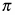] (θ is an element of the interval from 0 to 2). Homework Help ✎

1. csc θ =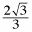2. sec θ = −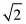3. tan θ is undefined

4. cot θ = −1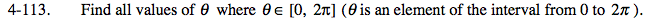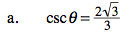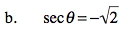Rewrite cscθ in terms of sinθ.

Flip the fraction on both sides.

Rationalize the denominator.

Simplify.

The sine function is positive in Quadrants I and II.

$\frac{1}{\text{sin}\theta}=\frac{2\sqrt{3}}{3}$

$\text{sin}\theta=\frac{3}{2\sqrt{3}}$

$\text{sin}\theta=\frac{3}{2\sqrt3}\left(\frac{\sqrt3}{\sqrt3}\right)$

$\text{sin}\theta=\frac{\sqrt3}{2}$

$\text{Therefore, }\theta=\frac{\pi}{3},\frac{2\pi}{3}.$

Use the same thinking process as in (a).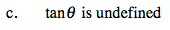$\frac{\text{sin}\theta}{\text{cos}\theta}$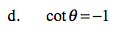Use the same thinking process as in (a).